Home
Hostname: page-component-99c86f546-z5d2w Total loading time: 0.327 Render date: 2021-12-03T08:26:56.250Z Has data issue: true Feature Flags: { "shouldUseShareProductTool": true, "shouldUseHypothesis": true, "isUnsiloEnabled": true, "metricsAbstractViews": false, "figures": true, "newCiteModal": false, "newCitedByModal": true, "newEcommerce": true, "newUsageEvents": true }Compositio Mathematica

# The$l$-parity conjecture for abelian varieties over function fields of characteristic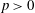$p>0$

Published online by Cambridge University Press:  10 March 2014

## Abstract

HTML view is not available for this content. However, as you have access to this content, a full PDF is available via the ‘Save PDF’ action button.

Let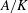$A/K$ be an abelian variety over a function field of characteristic$p>0$ and let$\ell$ be a prime number (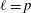$\ell =p$ allowed). We prove the following: the parity of the corank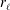$r_\ell$ of the$\ell$-discrete Selmer group of$A/K$ coincides with the parity of the order at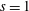$s=1$ of the Hasse–Weil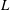$L$-function of$A/K$. We also prove the analogous parity result for pure$\ell$-adic sheaves endowed with a nice pairing and in particular for the congruence Zeta function of a projective smooth variety over a finite field. Finally, we prove that the full Birch and Swinnerton-Dyer conjecture is equivalent to the Artin–Tate conjecture.

## MSC classification

Type
Research Article
Information
Compositio Mathematica , April 2014 , pp. 507 - 522

## References

Abe, T. and Caro, D., Theory of weights in p-adic cohomology, Preprint (2013), arXiv:1303.0622.Google Scholar
Berthelot, P., Breen, L. and Messing, W., Théorie de Dieudonné Cristalline II, Lecture Notes in Mathematics, vol. 930 (Springer, 1982).CrossRefGoogle Scholar
Coates, J., Fukaya, T., Kato, K. and Sujatha, R., Root numbers, Selmer groups, and non-commutative Iwasawa theory, J. Algebraic Geom. 19 (2010), 1997.CrossRefGoogle Scholar
Česnavičius, K., The p-parity conjecture for elliptic curves with a p-isogeny. Preprint (2012),arXiv:1207.0431v2.Google Scholar
Crew, R., Finiteness theorems for the cohomology of an overconvergent isocrystal on a curve, Ann. Sci. Éc. Norm. Supér. (4) 31 (1998), 717763.CrossRefGoogle Scholar
Deligne, P., La conjecture de Weil : II, Publ. Math. Inst. Hautes Études Sci. 52 (1980), 137252.CrossRefGoogle Scholar
Dokchitser, T. and Dokchitser, V., Parity of ranks for elliptic curves with a cyclic isogeny, J. Number Theory 128 (2008), 662679.CrossRefGoogle Scholar
Dokchitser, T. and Dokchitser, V., Regulator constants and the parity conjecture, Inv. Math. 178 (2009), 2371.CrossRefGoogle Scholar
Dokchitser, T. and Dokchitser, V., On the Birch–Swinnerton-Dyer quotients modulo squares, Ann. of Math. (2) 172 (2010), 567596.CrossRefGoogle Scholar
Dokchitser, T. and Dokchitser, V., Root numbers and parity of ranks of elliptic curves, J. Reine Angew. Math. 2011 (658) (2011), 3964.CrossRefGoogle Scholar
Grothendieck, A. and Dieudonné, J., Eléments de géométrie algébrique (rédigés avec la collaboration de Jean Dieudonné) : II. Etude globale élémentaire de quelques classes de morphismes, Publ. Math. Inst. Hautes Études Sci. 8 (1961), 5222.Google Scholar
Etesse, J.-Y. and Le Stum, B., Fonctions L associés aux F-isocristaux surconvergents I. Interprétation cohomologique, Math. Ann. 296 (1993), 557576.CrossRefGoogle Scholar
Grothendieck, A., Le groupe de Brauer III, in Dix expos’es sur la cohomologie des schémas (North Holland, 1968).Google Scholar
Hartshorne, R., Algebraic geometry (Springer, New York, 1977), corrected 6th printing, 1993.CrossRefGoogle Scholar
Kato, K. and Trihan, F., On the conjecture of Birch and Swinnerton-Dyer in characteristic p > 0, Invent. Math. 153 (2003), 537592.CrossRefGoogle Scholar
Katz, N. and Messing, W., Some consequences for the Riemann hypothesis for varieties over finite fields, Invent. Math. 23 (1974), 7377.CrossRefGoogle Scholar
Kedlaya, K., Full faithfulness for overconvergent F-isocrystals, Geometric Aspects of Dwork Theory, vol. II (de Gruyter, Berlin, 2004), 819835.Google Scholar
Kedlaya, K., Fourier transform and p-adic Weil II, Compositio Math. 142 (2006), 14261450.CrossRefGoogle Scholar
Kiehl, R. and Weissauer, R., Weil conjectures, perverse sheaves and l-adic Fourier transform, Ergebnisse de Mathematik und ihere Grenzgebiete vol. 42 (Springer, Berlin, 2001).CrossRefGoogle Scholar
Kim, B. D., The parity conjecture for elliptic curves at supersingular reduction primes, Compositio Math. 143 (2007), 4772.CrossRefGoogle Scholar
Kim, B. D., The symmetric structure of the plus/minus Selmer groups of elliptic curves over totally real fields and the parity conjecture, J. Number Theory 129 (2009), 11491160.CrossRefGoogle Scholar
Lafforgue, L., Chtoucas de Drinfeld et correspondance de Langlands, Invent. Math. 147 (2002), 1242.CrossRefGoogle Scholar
Liu, Q., Algebraic geometry and arithmetic curves, Transl. by Reinie Ern. (English) Oxford Graduate Texts in Mathematics, vol. 6 (Oxford University Press, Oxford, 2006).Google Scholar
Liu, Q., Lorenzini, D. and Raynaud, M., On the Brauer group of a surface, Invent. Math. 159 (2005), 673676.CrossRefGoogle Scholar
Matsuda, S. and Trihan, F., Image directe supérieure et unipotence, J. Reine Angew. Math. 2004 (2004), 4754.CrossRefGoogle Scholar
Milne, J., Abelian varieties, in Proc. conf. on arithmetic geometry, Storrs, 1984 (Springer, New York, 1984), 103150.Google Scholar
Nekovář, J., On the parity of ranks of Selmer groups II, C. R. Acad. Sci. Paris, Ser. I 332 (2001), 99104.CrossRefGoogle Scholar
Nekovář, J., Selmer complexes, Astérisque 310 (2006).Google Scholar
Nekovář, J., On the parity of ranks of Selmer groups III, Doc. Math. 12 (2007), 243274; Erratum: Doc. Math. 14 (2009), 191–194.Google Scholar
Nekovář, J., On the parity of ranks of Selmer groups IV, Compositio Math. 145 (2009), 13511359; with an appendix by J.-P. Wintenberger.CrossRefGoogle Scholar
Nekovář, J., Some consequences of a formula of Mazur and Rubin for arithmetic local constants, Algebra Number Theory 7 (2013), 11011120.CrossRefGoogle Scholar
Oda, T., The first de Rham cohomology group and Dieudonné modules, Ann. Sci. Éc. Norm. Supér. (4) (1969), 63135.CrossRefGoogle Scholar
Schneider, P., Zur Vermutung von Birch und Swinnerton-Dyer über globalen Funktionskörpern., Math. Ann. 260 (1982), 495510.CrossRefGoogle Scholar
Tate, J., Conjectures on algebraic cycles in -adic cohomology, in Motives. Proceedings of the Summer Research Conference on Motives, University of Washington, Seattle, WA, USA, July 20–August 2, 1991, Proceedings of Symposia in Pure Mathematics, vol. 55, eds Jannsen, U. et al. (American Mathematical Society, Providence, RI, 1994), 7183; Pt. 1.Google Scholar
Trihan, F. and Wuthrich, C., Parity conjectures for elliptic curves over global fields of positive characteristic, Compositio Math. 147 (2011), 11051128.CrossRefGoogle Scholar
Ulmer, D., Curves and Jacobians over function fields, Preprint (2012),http://people.math.gatech.edu/∼ulmer/research/preprints/C.pdf.Google ScholarYou have Access
3
Cited by

# Send article to Kindle

Note you can select to send to either the @free.kindle.com or @kindle.com variations. ‘@free.kindle.com’ emails are free but can only be sent to your device when it is connected to wi-fi. ‘@kindle.com’ emails can be delivered even when you are not connected to wi-fi, but note that service fees apply.

Find out more about the Kindle Personal Document Service.

The$l$-parity conjecture for abelian varieties over function fields of characteristic$p>0$
Available formats
×

# Send article to Dropbox

To send this article to your Dropbox account, please select one or more formats and confirm that you agree to abide by our usage policies. If this is the first time you use this feature, you will be asked to authorise Cambridge Core to connect with your <service> account. Find out more about sending content to Dropbox.

The$l$-parity conjecture for abelian varieties over function fields of characteristic$p>0$
Available formats
×

# Send article to Google Drive

To send this article to your Google Drive account, please select one or more formats and confirm that you agree to abide by our usage policies. If this is the first time you use this feature, you will be asked to authorise Cambridge Core to connect with your <service> account. Find out more about sending content to Google Drive.

The$l$-parity conjecture for abelian varieties over function fields of characteristic$p>0$
Available formats
×
×